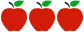### Grade 7: Operations with Rational Numbers

Rating:https://www.georgiastandards.org/Georgia-Standards/Frameworks/7th-Math-Unit-1.pdf

#### Common Core Standards

7.NS.1 - Apply and extend previous understandings of addition and subtraction to add and subtract rational numbers; represent addition and subtraction on a horizontal or vertical number line diagram.

7.NS.1a - Describe situations in which opposite quantities combine to make 0.

7.NS.1b - Understand p + q as the number located a distance |q| from p, in the positive or negative direction depending on whether q is positive or negative. Show that a number and its opposite have a sum of 0 (are additive inverses). Interpret sums of rational numbers by describing real-world contexts.

7.NS.1c - Understand subtraction of rational numbers as adding the additive inverse,p - q = p + (-q). Show that the distance between two rational numbers on the number line is the absolute value of their difference, and apply this principle in real-world contexts.

7.NS.1d - Apply properties of operations as strategies to add and subtract rational numbers.

7.NS.2 - Apply and extend previous understandings of multiplication and division and of fractions to multiply and divide rational numbers.

7.NS.2a - Understand that multiplication is extended from fractions to rational numbers by requiring that operations continue to satisfy the properties of operations, particularly the distributive property, leading to products such as (-1)(-1) = 1 and the rules for multiplying signed numbers. Interpret products of rational numbers by describing real-world contexts.

7.NS.2b - Understand that integers can be divided, provided that the divisor is not zero, and every quotient of integers (with non-zero divisor) is a rational number. If p and q are integers, then -(p/q) = (-p)/q = p/(-q). Interpret quotients of rational numbers by describing real-world contexts.

7.NS.2c  -Apply properties of operations as strategies to multiply and divide rational numbers.

7.NS.2d - Convert a rational number to a decimal using long division; know that the decimal form of a rational number terminates in Os or eventually repeats.

7.NS.3 - Solve real-world and mathematical problems involving the four operations with rational numbers.

Standards for Mathematical Practice

MP.1 Make sense of problems and persevere in solving them.

MP.2 Reason abstractly and quantitatively.

MP.3 Model with mathematics.

#### Description of Unit

The unit “Operations with Rational Numbers” from Georgia DOE focuses on understanding of operations of rational numbers. Students extend previous understanding of operating with fractions to adding, subtracting, multiplying, and dividing rational numbers. The unit is paced to 20-25 days and consists of 8 performance tasks. Each task is presented in a real-world context and allows students to develop their own strategies. Students model the operations of rational numbers in multiple ways and look for patterns in order to generate algorithms.

#### Cautions

Connecticut teachers should be aware that performance tasks do not identify which standards (content or practice) are being addressed. The unit does not include the following components:

• structures for implementing and completing performance tasks. There are no instructional notes (answer keys, rubrics, student work samples).
• differentiation or supports for students working above/below grade level, English language learners, or students with disabilities.
• a summative performance task or other type of summative assessment.
• There is only limited use of technology.